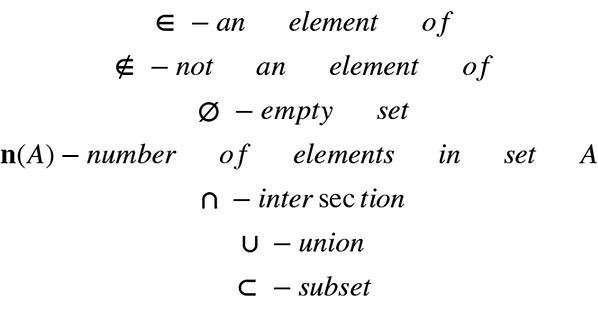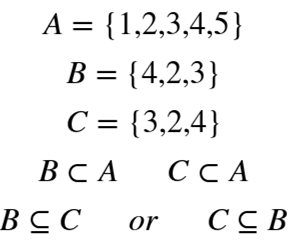#### 1.3 Set Language and Notation

Sets are used to convert raw data into categorized and sorted information. Since it is a visual tool, presenting data using sets can be useful as it is much easier to read.

The numbers or objects in the set are known as elements. The elements are placed within curly brackets to form a set. Before proceeding let's look at some of the symbols used when dealing with sets:Example 1: Now let's make a set. Let's assume this set should only contain odd numbers that are less 10. We can call this set A.

The odd numbers that are less that 10 are 1, 3, 5, 7 and 9. This means that there should be 5 elements in Set A. Putting them in the curly brackets will look like this: {1, 3, 5, 7, 9}. The elements in the set do not need to be ordered in a specific sequence; therefore, if there are 2 sets with the same elements but different sequence they are still considered equal: {1, 3, 5, 7, 9} = {3, 7, 1, 9, 5} = {5, 7, 1, 9, 3} etc.

Let's use the above symbols to describe set A:Since 7 is an element in the set we can write it as such:10 is not an element in the set:We have 4 element in set A:

Now let's assume that there is another set B. Set B should contain only prime numbers less than 10:

B = {2, 3, 5, 7}

If we say A U B, this means that elements in A and B are united to form a new set:

A U B = {1, 2, 3, 5, 7, 9}, it is important to note that the same elements present in both A and B are not written twice in the new set.

If we say A n B, this means that only the elements present in both sets are considered:

A n B = {3, 5, 7}.

When dealing with more than 1 set, it is important to determine the universal set. The universal set contains all possible elements considered for a particular problem. It could be a set containing all elements combined from all the other sets plus the elements that were not even considered.

We can take our universal set in this case to be all numbers between 1 and 10:We are using the script E to denote the universal set in this case. Instead of the script E, U can also be used.

Now that we established what the universal set is, we can determine the complement of set A and B. A complement of a particular set contains all elements in the universal set except for the elements in that set.

The complement of set A is:

A' = {2, 4, 6, 8, 10}

The complement of set B is:

B' = {1, 4, 6, 8, 9, 10}

Finally, let's look at what subsets are. A subset is a set that is a part of a larger set. For example assume that Set A contains all peppers. Set B contains jalapenos. Set B is a subset of set A since jalapenos are a type of pepper.

A subset can either be a proper subset or not a proper subset. Let's look at an example to understand this:

Example 2:
Assume:

Set A = {1, 2, 3, 4, 5 }

Set B = { 4, 2, 3 }
Set C = {3, 2, 4 }

As you can see both B and C are subsets of A because the elements 2, 3, and 4 are also present in Set A. However Set B and C are not equal to A because set A has additional elements not present in B or C. Therefore B and C are proper subsets of set A.

Set C is also a subset of set B; however, in this case B and C have the same elements and are equal. Therefore, set C is not a proper subset of set B and vice versa.Not a proper subset symbol is basically the symbol for subset with a line under it. For a proper subset, the subset must always be on the closed side of the symbol. However, for not a proper subset it does not matter since both sets are equal.